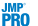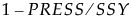<Cross Validation Method> and Method = <Method Specification>
Cross Validation ReportWhen the Standardize X option is selected, the standardization is applied once to the entire data table. It is not reapplied to the individual training sets. However, when any combination of the Centering or Scaling options are selected, this combination of selections is applied to each cross validation training set. Cross validation proceeds by using the training sets, which are individually centered and scaled if these options are selected.
Test statistic for the van der Voet test, which tests whether models with different numbers of extracted factors differ significantly from the optimum model. The null hypothesis for each van der Voet T2 test states that the model based on the corresponding number of factors does not differ from the optimum model. The alternative hypothesis is that the model does differ from the optimum model. For more details, see van der Voet T2.
p-value for the van der Voet T2 test. For more details, see van der Voet T2.
Q2
Here PRESSi and SSYi correspond to their values for i factors.
R2X
R2Y
Interpretation of Q2 and Cumulative R2Y
The statistics Q2 and Cumulative R2Y both measure the predictive ability of the model, but in different ways.
 • Cumulative R2Y increases as the number of factors increases. This is because, as factors are added to the model, more variation is explained.
 • Q2 tends to increase and then decrease, or at least discontinue increasing, as the number of factors increases. This is because, as more factors are added, the model becomes tuned to the training set and does not generalize well to new data, causing the PRESS statistic to decrease.
Analysis of Q2 and Cumulative R2Y provides an alternative to using the van der Voet test for determining how many factors to include in your model. Select a number of factors for which Q2 is large and has not started decreasing. You also want Cumulative R2Y to be large.
Cumulative R2Y and Q2 for Penta.jmp shows plots of Cumulative R2Y and Q2 against the number of factors for the Penta.jmp data table, using Leave-One-Out as the validation method. Cumulative R2Y increases and levels off for about four factors. The statistic Q2 is largest for two factors and then begins to level off. The plot suggests that a model with two factors will explain a large portion of the variation in Y without overfitting the data.
Cumulative R2Y and Q2 for Penta.jmp
For a specified number of factors, a, Root Mean PRESS is calculated as follows:
 1 Fit a model with a factors to each training set.
 2 Apply the resulting prediction formula to the observations in the validation set.
 3 For each Y:
 – For each validation set, compute the squared difference between each observed validation set value and its predicted value (the squared prediction error).
 – Sum these means and, in the case of more than one validation set, divide their sum by the number of validation sets minus one. This is the PRESS statistic for the given Y.
 4 Root Mean PRESS for a factors is the square root of the average of the PRESS values across all responses.
 5
The statistic Q2 is defined as. The PRESS statistic is the predicted error sum of squares averaged across all responses for the model developed based on the training data, but evaluated on the validation set. The value of SSY is the sum of squares for Y averaged across all responses and based on the observations in the validation set.
The statistic Q2 in the Cross Validation report is computed in the following ways, depending on the selected Validation Method:
Q2 is the average of the valuescomputed for the validation sets based on the models constructed by leaving out one observation at a time.
Q2 is the average of the valuescomputed for the validation sets based on the K models constructed by leaving out each of the K folds.
Q2 is the value ofcomputed for the validation set based on the model constructed using the single set of training data.
Calculation of R2X and R2Y When Validation Is Used
The statistics R2X and R2Y in the Cross Validation report are computed in the following ways, depending on the selected Validation Method:
Note: For all of these computations, R2Y is calculated analogously.
R2X is the average of the Percent Variation Explained for X Effects for the models constructed by leaving out one observation at a time.
R2X is the average of the Percent Variation Explained for X Effects for the K models constructed by leaving out each fold.
R2X is the Percent Variation Explained for X Effects for the model constructed using the training data.

Help created on 9/19/2017